## Fundamental Forms

There are three types of so-called fundamental forms. The most important are the first and second (since the third can be expressed in terms of these). The fundamental forms are extremely important and useful in determining the metric properties of a surface, such as Line Element, Area Element, Normal Curvature, Gaussian Curvature, and Mean Curvature. Let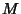be a Regular Surface withpoints on the Tangent Spaceof. Then the first fundamental form is the Inner Product of tangent vectors,(1)

For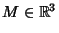, the second fundamental form is the symmetric bilinear form on the Tangent Space,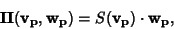(2)

where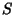is the Shape Operator. The third fundamental form is given by(3)

The first and second fundamental forms satisfy(4)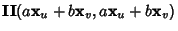(5)

and so their ratio is simply the Normal Curvature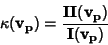(6)

for any nonzero Tangent Vector. The third fundamental form is given in terms of the first and second forms by(7)

where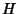is the Mean Curvature andis the Gaussian Curvature.

The first fundamental form (or Line Element) is given explicitly by the Riemannian Metric(8)

It determines the Arc Length of a curve on a surface. The coefficients are given by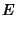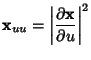(9)(10)(11)

The coefficients are also denoted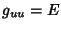,, and. In Curvilinear Coordinates (where), the quantities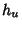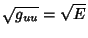(12)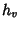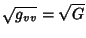(13)

are called Scale Factors.

The second fundamental form is given explicitly by(14)

where(15)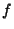(16)(17)

andare the Direction Cosines of the surface normal. The second fundamental form can also be written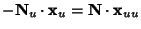(18)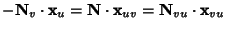(19)(20)

where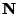is the Normal Vector, or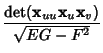(21)(22)(23)

See also Arc Length, Area Element, Gaussian Curvature, Geodesic, Kähler Manifold, Line of Curvature, Line Element, Mean Curvature, Normal Curvature, Riemannian Metric, Scale Factor, Weingarten Equations

References

Gray, A. The Three Fundamental Forms.'' §14.6 in Modern Differential Geometry of Curves and Surfaces. Boca Raton, FL: CRC Press, pp. 251-255, 259-260, 275-276, and 282-291, 1993.